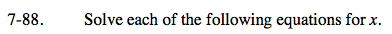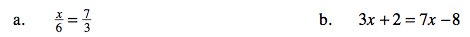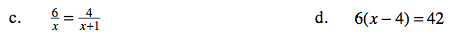### Home > CAAC > Chapter 7 > Lesson 7.3.1 > Problem7-88

7-88.Multiply both sides by 18.

$(18)\frac{x}{6}=\frac{7}{3}(18)$

3x = 42

Divide both sides by 3.

x = 14

Don't forget to check your solution!

Subtract 3x from both sides.

3x + 2 = 7x − 8
−3x = −3x
2 = 4x − 8

2 = 4x − 8
+8 = +8
10 = 4x

Divide by 4.

$x=\frac{10}{4}=\frac{5}{2}=2.5$